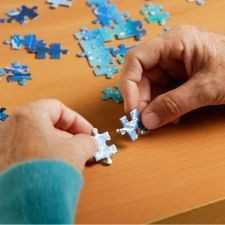Brainy Puzzles
Puzzle Solvers: Interesting brainy puzzles shared by fellow puzzlers
383 Members
Join this group to post and comment.Kaustubh Katdare • Jul 25, 2008

# Now crack this simple numbers puzzle

How many numbers is it possible to form with only 3 digits (excluding zero) and what is the total of all these numbers?

[Say no to Google, get wise!]

Any answer posted without detailed explanation is invalid 😉 !bayazidahmed • Aug 7, 2008
Answer to the first question: 9*9*9 = 729

Answer to the second question: There are many ways to solve this. I'll post the simplest solution I found.
The median in this case is 555 and this can be said as mean also in this case.
Total = 555 * 729 = 404595mahul • Aug 8, 2008
729 numbers in all as bayazidahmed says. As for each places we have nine options, no: of ways to create the numbers = 9*9*9 = 729.

However my second answer would differ. There would be 81 instances of each number appearing in the units, tens and hundreds place respectively. Hence the sum would basically be a summation of

81*111+81*222+81*333........+81*999 = 81*(111+222+333.....+999)
= 81*5550 = 449550bayazidahmed • Aug 9, 2008
why 81*111+81*222+81*333........+81*999?

using your logic, I think it should be
81*155 + 81*255 + ... + 81*955 = 81*(155 + 255 + ... + 955) = 404595mahul • Aug 9, 2008
I did not get your reasoning. So I better post mine.

Since there are 81 instances of say '1' appearing in the units, tens and hundreds place respectively, I have clubbed them together into 81 instances of '111'. Similar is the case with the rest of the 9 digits.bayazidahmed • Aug 10, 2008
believe me i am too tired to post mine after a hectic day.
Why are you multiplying with 111?

Alternatively, The_Big_K give the correct answer!anuragh27crony • Aug 11, 2008
explained Reasoning for Mahul.......

Since 1 can be on ones position....81 times..and all those count to--- 81*1
Since 1 can be on Tens postion....81 times...and all those count to---81*10
Since 1 can be on Hundered's postion...81 times...all those count to--81*100

so on combining these 81*(1+10+100) --> 81*111

Similarly for remaining Numbers......bayazidahmed • Aug 12, 2008
*sigh*

Lets analyze your first line
Since 1 can be on ones position....81 times..and all those count to--- 81*1??
you mean to say the sum off all the numbers that have 1 in units place is 81*1? Let me write and sum it for you,
111 + 121 + 131 + 141 + 151 + 161 + 171 + 181 + 191 + 211 + 221 + 231 + 241 + 251 + 261 + 271 + 281 + 291 + 311 + 321 + 331 + 341 + 351 + 361 + 371 + 381 + 391 + 411 + 421 + 431 + 441 + 451 + 461 + 471 + 481 + 491 + 511 + 521 + 531 + 541 + 551 + 561 + 571 + 581 + 591 + 611 + 621 + 631 + 641 + 651 + 661 + 671 + 681 + 691 + 711 + 721 + 731 + 741 + 751 + 761 + 771 + 781 + 791 + 811 + 821 + 831 + 841 + 851 + 861 + 871 + 881 + 891 + 911 + 921 + 931 + 941 + 951 + 961 + 971 + 981 + 991 = 44631
It certainly doesnt come to 81*1!

Since 1 can be on Tens postion....81 - 9(we counted this with ones) = 72 times
Since 1 can be on Hundered's postion...81 - 9(we counted this with ones) - 8(we counted this with hundreds) = 64 times

and if i keep on continuing this, it will be a nightmare for me to keep the numbers from not being repeated.

In btw, I created an excel file just now, deleted all the numbers having a 0 and did a quick sum and the result came to 404595 😎anuragh27crony • Aug 12, 2008
srry dude for not being clear......
i think this would be more clear than earlier

100*x+10*Y+1*1 --there can be such 81 numbers

count only the ones position in such numbers with number 1..........so they count to 81*1

similarly 100*x+10*1+y*1-- there can be such 81 numbers...count only tens position in such numbers with 1 ---so they count to 81*10

similarly 100*1+10*x+y*1-- there can be such 81 numbers...count only hundereds position with 1 ---so they count to 81*100

so same applicable for other numbers at different positions as wellbayazidahmed • Aug 17, 2008
*huff*

I cannot dwell anymore, verify it for yourself. I tried in excel, its coming for me 404595, not 449550!